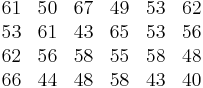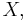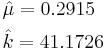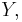# Gamma Distribution Example

(Redirected from Example: Gamma Distribution)

This example appears in the Life Data Analysis Reference book.

24 units were reliability tested, and the following life test data were obtained:$\begin{matrix} \text{61} & \text{50} & \text{67} & \text{49} & \text{53} & \text{62} \\ \text{53} & \text{61} & \text{43} & \text{65} & \text{53} & \text{56} \\ \text{62} & \text{56} & \text{58} & \text{55} & \text{58} & \text{48} \\ \text{66} & \text{44} & \text{48} & \text{58} & \text{43} & \text{40} \\ \end{matrix}\,\!$

Fitting the gamma distribution to this data, using maximum likelihood as the analysis method, gives the following parameters:\begin{align} & \hat{\mu }= 7.72E-02 \\ & \hat{k}= 50.4908 \end{align}\,\!

Using rank regression on$X,\,\!$ the estimated parameters are:\begin{align} & \hat{\mu }= 0.2915 \\ & \hat{k}= 41.1726 \end{align}\,\!

Using rank regression on$Y,\,\!$ the estimated parameters are:\begin{align} & \hat{\mu }= 0.2915 \\ & \hat{k}= 41.1726 \end{align}\,\!